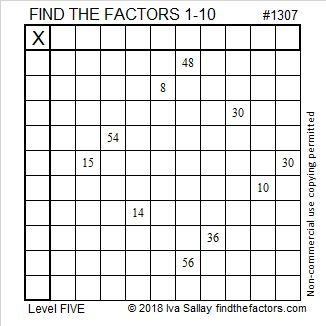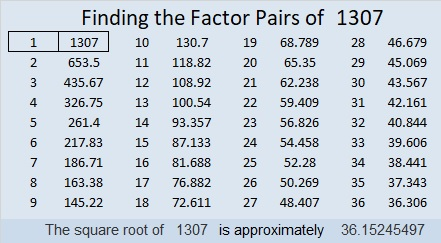# 1307 and Level 5

This puzzle shows 10 of the 100 products in a 10 × 10 multiplication table. Can you figure out where to put the factors? There’s only one way that works!Print the puzzles or type the solution in this excel file: 10-factors-1302-1310

Here are some facts about the number 1307:

• 1307 is a prime number.
• Prime factorization: 1307 is prime.
• The exponent of prime number 1307 is 1. Adding 1 to that exponent we get (1 + 1) = 2. Therefore 1307 has exactly 2 factors.
• Factors of 1307: 1, 1307
• Factor pairs: 1307 = 1 × 1307
• 1307 has no square factors that allow its square root to be simplified. √1307 ≈ 36.15245

How do we know that 1307 is a prime number? If 1307 were not a prime number, then it would be divisible by at least one prime number less than or equal to √1307 ≈ 36.2. Since 1307 cannot be divided evenly by 2, 3, 5, 7, 11, 13, 17, 19, 23, 29 or 31, we know that 1307 is a prime number.1307 is palindrome 797 in BASE 13 because 7(13²) + 9(13) + 7(1) = 1307

This site uses Akismet to reduce spam. Learn how your comment data is processed.